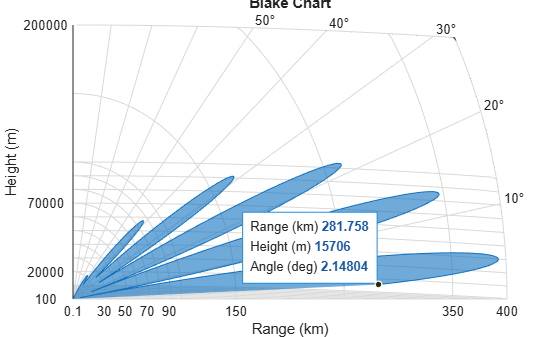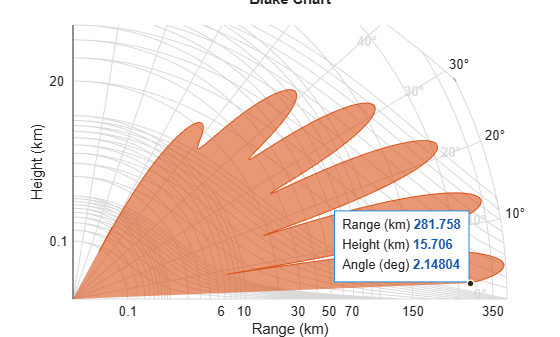Vertical coverage diagram

## Syntax

``[vcp,vcpangles] = radarvcd(freq,rfs,anht)``
``[vcp,vcpangles] = radarvcd(___,Name,Value)``
``radarvcd(___)``

## Description

example

````[vcp,vcpangles] = radarvcd(freq,rfs,anht)` calculates the vertical coverage pattern of a narrowband radar antenna. The Vertical Coverage Pattern is the range of the radar `vcp` as a function of elevation angle `vcpangles`. The vertical coverage pattern depends on three parameters: maximum free-space detection range of the radar `rfs`, the radar frequency `freq`, and the antenna height `anht`.```

example

````[vcp,vcpangles] = radarvcd(___,Name,Value)` allows you to specify additional input parameters using name-value arguments. You can specify multiple name-value arguments in any order.```

example

````radarvcd(___)` displays the vertical coverage diagram for a radar system. The plot is the locus of points of maximum radar range as a function of target elevation. This plot is also known as the Blake chart. To create this chart, `radarvcd` invokes the function `blakechart` using default parameters. To produce a Blake chart with different parameters, first call `radarvcd` to obtain `vcp` and `vcpangles`. Then, call `blakechart` with user-specified parameters. This syntax can use any of the previous syntaxes.```

## Examples

collapse all

Set the frequency to 100 MHz, the antenna height to 10 m, and the free-space range to 200 km. The antenna pattern, surface roughness, antenna tilt angle, and field polarization assume their default values as specified in the `AntennaPattern`, `SurfaceRoughness`, `TiltAngle`, and `Polarization` properties.

Obtain an array of vertical coverage pattern values and angles.

```freq = 100e6; ant_height = 10; rng_fs = 200; [vcp,vcpangles] = radarvcd(freq,rng_fs,ant_height);```

To see the vertical coverage pattern, omit the output arguments.

`radarvcd(freq,rng_fs,ant_height);`Set the frequency to 100 MHz, the antenna height to 10 m, and the free-space range to 200 km. The antenna pattern is a sinc function with 45° half-power width. The surface height standard deviation is set to $1/2\sqrt{2}$ m. The antenna tilt angle is set to 0°, and the field polarization is horizontal.

```pat_angles = linspace(-90,90,361)'; freq = 100e6; ntn = phased.SincAntennaElement('Beamwidth',45); pat = ntn(freq,pat_angles'); ant_height = 10; rng_fs = 200; tilt_ang = 0; [vcp,vcpangles] = radarvcd(freq,rng_fs,ant_height,... 'RangeUnit','km','HeightUnit','m',... 'AntennaPattern',pat,... 'PatternAngles',pat_angles,... 'TiltAngle',tilt_ang,'SurfaceHeightStandardDeviation',1/(2*sqrt(2)));```

Call `radarvcd` with no output arguments to display the vertical coverage pattern.

```radarvcd(freq,rng_fs,ant_height,... 'RangeUnit','km','HeightUnit','m',... 'AntennaPattern',pat,... 'PatternAngles',pat_angles,... 'TiltAngle',tilt_ang,'SurfaceHeightStandardDeviation',1/(2*sqrt(2)));```Alternatively, use the `radarvcd` output arguments and the `blakechart` function to display the vertical coverage pattern to a maximum range of 400 km and a maximum height of 50 km. Customize the Blake chart by changing the color.

```blakechart(vcp,vcpangles,400,50, ... 'FaceColor',[0.8500 0.3250 0.0980],'EdgeColor',[0.8500 0.3250 0.0980])```Plot the range-height-angle curve (Blake chart) for a radar with a user-specified antenna pattern.

Define a sinc-function antenna pattern with a half-power beamwidth of 90 degrees. The radar transmits at 100 MHz.

```pat_angles = linspace(-90,90,361)'; freq = 100e6; ntn = phased.SincAntennaElement('Beamwidth',90); pat = ntn(freq,pat_angles');```

Specify a free-space range of 200 km. The antenna height is 10 meters, the antenna tilt angle is zero degrees, and the surface roughness is one meter.

```rng_fs = 200; ant_height = 10; tilt_ang = 0; surf_roughness = 1;```

```radarvcd(freq,rng_fs,ant_height,... 'RangeUnit','km','HeightUnit','m',... 'AntennaPattern',pat,... 'PatternAngles',pat_angles,... 'TiltAngle',tilt_ang,... 'SurfaceHeightStandardDeviation',surf_roughness/(2*sqrt(2)));```## Input Arguments

collapse all

Radar frequency, specified as a real-valued scalar less than 10 GHz (1010 Hz).

Example: 100e6

Data Types: `double`

Free-space range, specified as a positive scalar or vector. `rfs` is the calculated or assumed free-space range for a target or for a one-way RF system at which the field strength would have a specified value. Range units are set by the `RangeUnit` name-value argument.

Example: 100e3

Data Types: `double`

Radar antenna height, specified as a real-valued scalar. The height is referenced to the surface. Height units are set by the `HeightUnit` name-value argument.

Example: 10

Data Types: `double`

### Name-Value Arguments

Specify optional pairs of arguments as `Name1=Value1,...,NameN=ValueN`, where `Name` is the argument name and `Value` is the corresponding value. Name-value arguments must appear after other arguments, but the order of the pairs does not matter.

Before R2021a, use commas to separate each name and value, and enclose `Name` in quotes.

Example: `'HeightUnit','km'`

Radar range units denoting kilometers, nautical miles, miles, feet, meters, or kilofeet. This argument specifies the units for the free-space range argument `rfs` and the output vertical coverage pattern `vcp`.

Example: `'mi'`

Data Types: `char`

Antenna height units denoting meters, nautical miles, miles, kilometers, feet, or kilofeet. This argument specifies the units for the antenna height `anht` and the `'SurfaceRoughness'` argument.

Example: `'m'`

Data Types: `char`

Transmitted wave polarization, specified as `'H'` for horizontal polarization or `'V'` for vertical polarization.

Example: `'V'`

Data Types: `char`

Complex permittivity (dielectric constant) of the reflecting surface, specified as a complex-valued scalar. The default value of this argument depends on the value of `freq`. `radarvcd` uses a seawater model that is valid for frequencies up to 10 GHz.

Example: 70

Data Types: `double`
Complex Number Support: Yes

Standard deviation of surface height, specified as a nonnegative real-valued scalar. A value of `0` indicates a smooth surface. Use `'HeightUnit'` to specify the units of height.

The surface height standard deviation relates to the crest-to-trough "surface roughness" height through

Surface roughness = 2 × √2 × Surface height standard deviation.

Example: 2

Data Types: `double`

Surface slope in degrees, specified as a nonnegative scalar. This value is expected to be 1.4 times the RMS surface slope. Given the condition that

2 × GRAZ/β0 < 1,

where GRAZ is the grazing angle of the geometry specified in degrees and β0 is the surface slope, the effective surface height standard deviation in meters is calculated as

Effective HGTSD = HGTSD × (2 × GRAZ/β0)1/5.

This calculation better accounts for shadowing. Otherwise, the effective height standard deviation is equal to HGTSD. This argument defaults to `0`, indicating a smooth surface.

Data Types: `double`

Surface vegetation type, specified as `'Trees'`, `'Weeds'`, and `'Brush'` are assumed to be dense vegetation. `'Grass'` is assumed to be thin grass. Use this argument when using the function on surfaces different from the sea.

Half-power elevation beamwidth in degrees, specified as a scalar between 0° and 90°. The elevation beamwidth is used in the calculation of a `sinc` antenna pattern. The default antenna pattern is symmetric with respect to the beam maximum and is of the form sin(u)/u. The parameter u is given by u = k sin(θ), where θ is the elevation angle in radians and k is given by k = x0 / sin(π × ELBW/360), where ELBW is the half-power elevation beamwidth and x0 ≈ 1.3915573 is a solution of sin(x) = x/√2.

Data Types: `double`

Antenna elevation pattern, specified as a real-valued column vector. Values for `'AntennaPattern'` must be specified together with values for `'PatternAngles'`. Both vectors must have the same size. If both an antenna pattern and an elevation beamwidth are specified, `radarvcd` uses the antenna pattern and ignores the elevation beamwidth value. This argument defaults to a sinc antenna pattern.

Example: `cosd([–90:90])`

Data Types: `double`

Antenna pattern elevation angles specified as a real-valued column vector. The size of the vector specified by `PatternAngles` must be the same as that specified by `AntennaPattern`. Angle units are expressed in degrees and must lie between –90° and 90°. In general, the antenna pattern should fill the whole range from –90° to 90° for the coverage to be computed properly.

Example: `[-90:90]`

Data Types: `double`

Antenna tilt angle, specified as a real-valued scalar. The tilt angle is the elevation angle of the antenna with respect to the surface. Angle units are expressed in degrees.

Example: `10`

Data Types: `double`

Effective Earth radius in meters, specified as a positive scalar. The effective Earth radius is an approximation used for modeling refraction effects in the troposphere. The default value calculates the effective Earth radius using a refraction gradient of `-39e-9`, which results in approximately `4/3` of the real Earth radius.

Data Types: `double`

Maximum elevation angle, specified as a real-valued scalar. The maximum elevation angle is the largest angle for which the vertical coverage pattern is calculated. Angle units are expressed in degrees.

Example: 70

Data Types: `double`

Minimum elevation angle, specified as a real-valued scalar. The minimum elevation angle is the smallest angle for which the vertical coverage pattern is calculated. Angle units are expressed in degrees.

Example: 10

Data Types: `double`

Elevation angle increment, specified as a positive scalar in degrees. The elevation vector goes from the minimum value specified in `MinElevation` and the maximum value specified in `MaxElevation` in increments of `ElevationStepSize`. The default value of this argument is given by

Δ = 885.6/(π × fMHz × ha,ft),

where fMHz is the frequency in MHz and ha,ft is the antenna height in feet.

Data Types: `double`

## Output Arguments

collapse all

Vertical coverage pattern, returned as a real-valued column vector or matrix. The vertical coverage pattern is the actual maximum range of the radar. Each row of the vertical coverage pattern corresponds to one of the angles returned in `vcpangles` The columns of `vcp` correspond to the ranges specified in `rfs`.

Vertical coverage pattern angles, returned as a column vector. The angles range from –90° to 90°. Each entry of `vcpangles` specifies the elevation angle at which the vertical coverage pattern is measured.